# How to use rectangles in a custom legend

This post describes how to build customize legends in Matplotlib to include rectangles in the handles when they are not obtained by default.

## Problem

For various reasons you may want to add a legend with handles that consist of squares or rectangles. For some plots, such as the ones obtained with `plt.fill_between()` the legend handle is going to be a rectangle by default (see this example).

However, for other types of charts, you will have to build them up from scratch.

## Example

Let's see this problem live with a scatterplot:

``````import palmerpenguins

import matplotlib.pyplot as plt
import numpy as np

from matplotlib.patches import Patch``````

``penguins = palmerpenguins.load_penguins().dropna()``
``````FLIPPER_LENGTH = penguins["flipper_length_mm"].values
BILL_LENGTH = penguins["bill_length_mm"].values

SPECIES = penguins["species"].values
SPECIES_ = np.unique(SPECIES)

COLORS = ["#1B9E77", "#D95F02", "#7570B3"]``````

The following code is extracted from the mentioned post on custom legends. Let's see what is the default legend we get for a scatterplot

``````fig, ax = plt.subplots(figsize=(9, 6))
for species, color in zip(SPECIES_, COLORS):
idxs = np.where(SPECIES == species)
ax.scatter(
FLIPPER_LENGTH[idxs], BILL_LENGTH[idxs], label=species,
s=50, color=color, alpha=0.7
)
legend = ax.legend();``````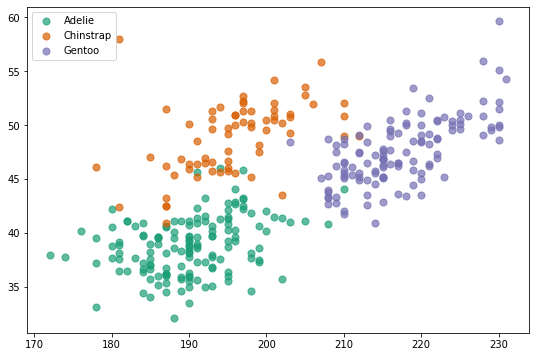Here we are, a scatterplot with circles used in the legend. How to use rectangles instead?

## Using rectangles in legend

Let's see how we can override this default behavior and use a rectangle instead. The following function is created to make it simpler to replicate the same plot several times.

``````def scatterplot():
fig, ax = plt.subplots(figsize=(9, 6))
for species, color in zip(SPECIES_, COLORS):
idxs = np.where(SPECIES == species)
ax.scatter(
FLIPPER_LENGTH[idxs], BILL_LENGTH[idxs],
s=50, color=color, alpha=0.7
)
return fig, ax``````

Let's generate the chart and create the handles for the legend. This is as simple as using `matplotlib.patches.Patch`.

``````fig, ax = scatterplot()

handles = [
Patch(facecolor=color, label=label)
for label, color in zip(SPECIES_, COLORS)
]

ax.legend(handles=handles);``````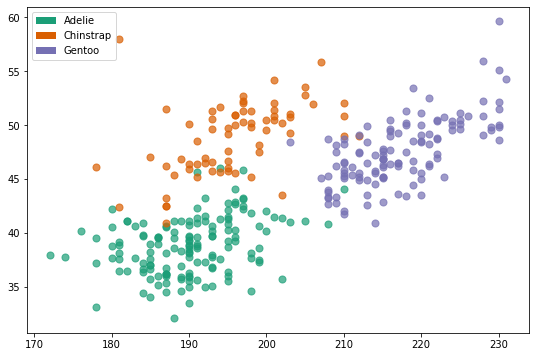## Customizing the rectangle

It's also possible to remove the fill and just leave the color of the borders.

``````fig, ax = scatterplot()

handles = [
Patch(edgecolor=color, label=label, fill=False)
for label, color in zip(SPECIES_, COLORS)
]

ax.legend(handles=handles);``````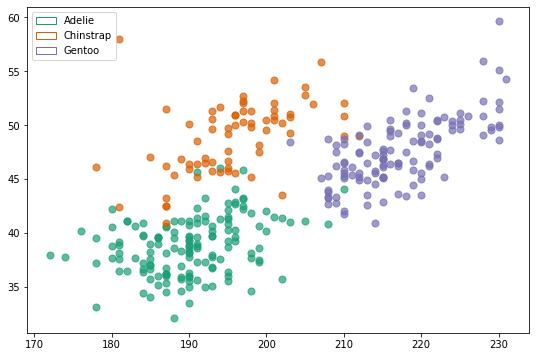Or use one color for the fill, and another for the border:

``````fig, ax = scatterplot()

handles = [
Patch(facecolor=color, edgecolor="k", label=label)
for label, color in zip(SPECIES_, COLORS)
]

ax.legend(handles=handles);``````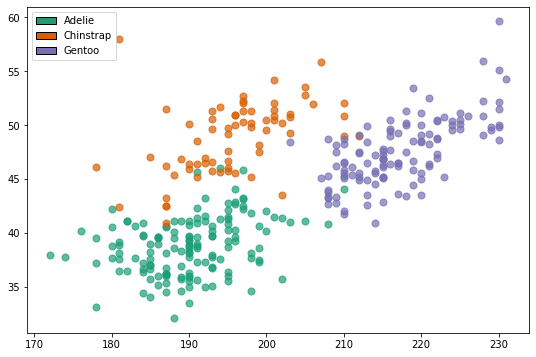And if you want to make them squared, you only need to set both `handlelength` and `handleheight` to the same value when creating the legend.

``````fig, ax = scatterplot()

handles = [
Patch(facecolor=color, edgecolor="k", label=label, alpha=0.7)
for label, color in zip(SPECIES_, COLORS)
]

legend = ax.legend(handles=handles, handlelength=1.4, handleheight=1.4);``````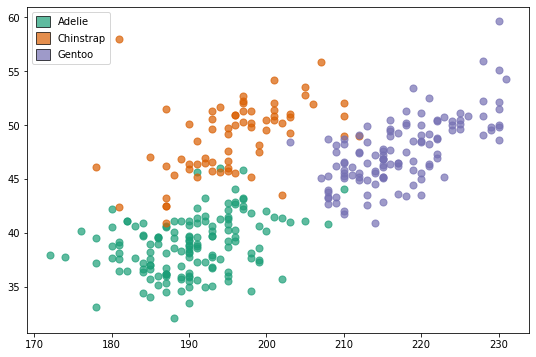## Contact & Edit

👋 This document is a work by Yan Holtz. Any feedback is highly encouraged. You can fill an issue on Github, drop me a message onTwitter, or send an email pasting `yan.holtz.data` with `gmail.com`.